# Useful Text Functions: LEFT, RIGHT, and MID

In last week’s post, I started my discussion of text functions. If you don’t remember what a “text function” is, check out last week’s post to refresh your memoryToday’s functions are a little more complicated, but still very easy to grasp. I want to tell you about the LEFT, RIGHT, and MID functions. These let you take little pieces, or “snippets” if you will, from your text.

## The LEFT Function

The LEFT function takes a piece of your text, starting from the left-hand side. You just need to tell Excel where your text is, and how many characters to count.

Let’s try an example. Say we have the text “Savvy Spreadsheets” in cell B2. If we want just the “Savvy” portion of the text, we can use the LEFT function. If you type “=LEFT(B2,5)” in cell C2, you’re telling Excel to go the the text in B2, and take 5 characters starting from the left (or the beginning of the string).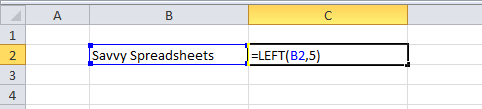Here’s the result.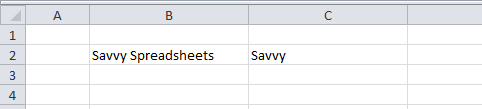## The RIGHT Function

The RIGHT function, as you probably guessed, is the opposite of the LEFT function. Instead of counting characters from the left-hand side, RIGHT counts starting at the right-hand side (the end of the text).

Let’s use the RIGHT function to grab “Spreadsheets” from “Savvy Spreadsheets.” Since “Spreadsheets” is 12 characters long, our formula would be “=RIGHT(B2,12).”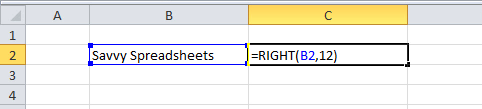The result would look like this.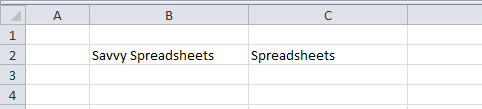## The MID Function

Just like LEFT and RIGHT, MID takes a portion of your text. But instead of starting at the beginning or end, it starts somewhere in the middle. There are three parts to this function. First you select your text, then you enter the number of characters from which Excel should start counting, and then you enter the number of characters it should count.

Using the MID function, let’s grab a piece in the middle of “Savvy Spreadsheets,” say the “Spread” part. Before we start typing, let’s count the number of characters in our string. In “Savvy Spreadsheets” there are 18 characters (the space between “Savvy” and “Spreadsheets” is also considered a character). There are 6 characters before “Spread” (including the space), so Excel needs to start counting at 7. Then, it needs to count 6 characters since that’s how long “Spread” is. Our formula would be “=MID(B2,7,6).”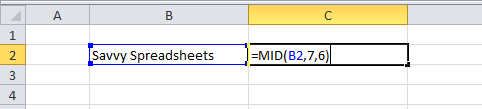And here is the result.LEFT, RIGHT, and MID are really simple and intuitive functions once you get the hang of them. When you have many rows of text with similar characteristics, you can do some serious data manipulation with these functions!